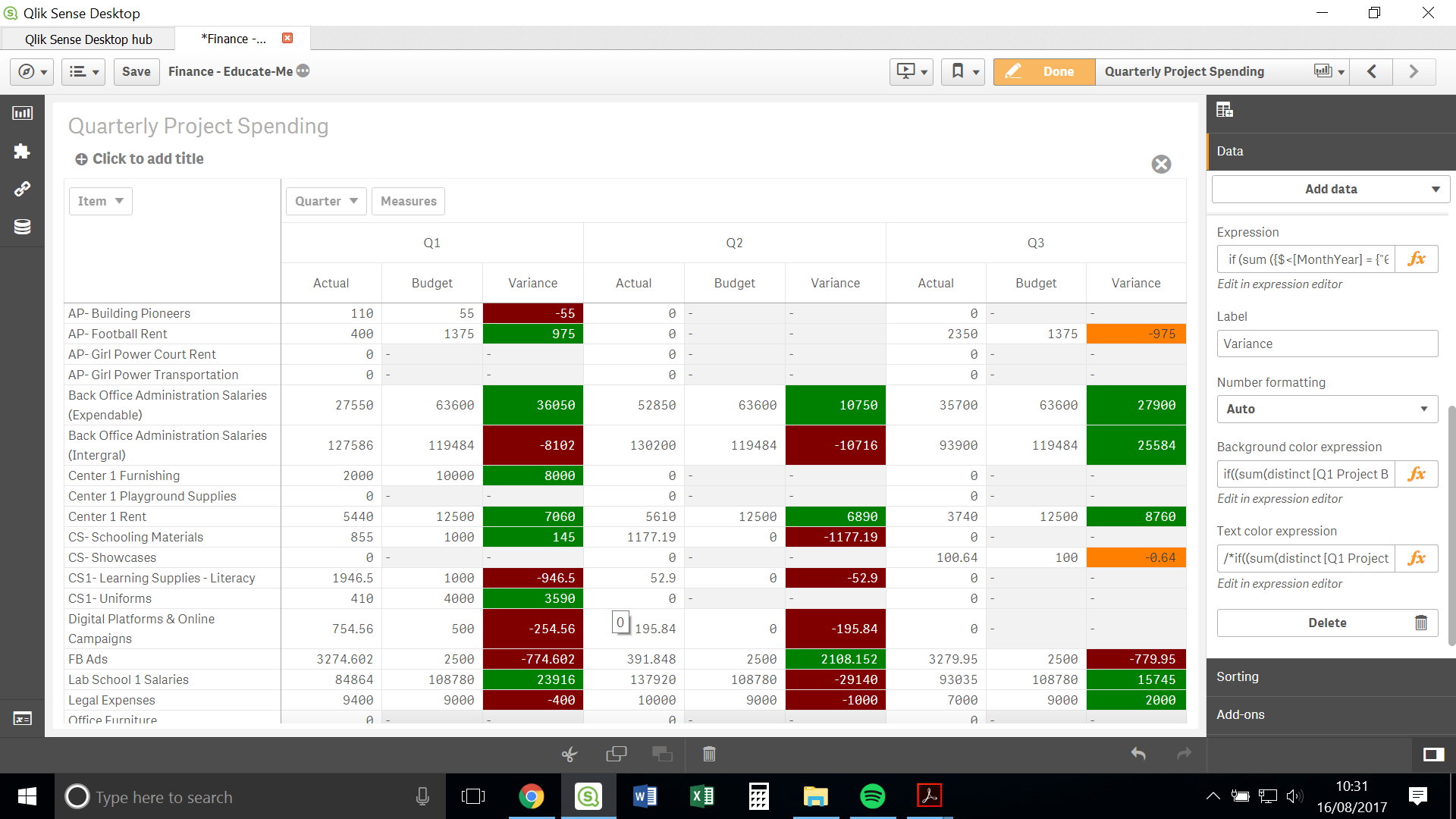Announcements
You can succeed best and quickest by helping others to succeed. Join the conversation.
cancel
Showing results for
Did you mean:Not applicable

## Colour Expression Inconsistent

Hello all,

Please can you help me with the following.I have the following expression:

if((sum(distinct [Q1 Project Budget])- sum({\$<Quarter = {Q1}>}[Project Expenditure Amount]))<0, red(),

if((sum(distinct [Q1 Project Budget])- sum({\$<Quarter = {Q1}>}[Project Expenditure Amount]))< sum(distinct [Q1 Project Budget]) , Green(),

if((sum(distinct [Q1 Project Budget])- sum({\$<Quarter = {Q1}>}[Project Expenditure Amount]))=0, RGB(255, 128, 0),

if((sum(distinct [Q2 Project Budget])- sum({\$<Quarter = {Q2}>}[Project Expenditure Amount]))<0, red(),

if((sum(distinct [Q2 Project Budget])- sum({\$<Quarter = {Q2}>}[Project Expenditure Amount]))< sum(distinct [Q2 Project Budget]) , Green(),

if((sum(distinct [Q2 Project Budget])- sum({\$<Quarter = {Q2}>}[Project Expenditure Amount]))=0, RGB(255, 128, 0),

if((sum(distinct [Q3 Project Budget])- sum({\$<Quarter={Q3}>}[Project Expenditure Amount]))<0, red(),

if((sum(distinct [Q3 Project Budget])- sum({\$<Quarter={Q3}>}[Project Expenditure Amount]))<sum(distinct [Q3 Project Budget]), Green(),

if((sum(distinct [Q3 Project Budget])- sum({\$<Quarter={Q3}>}[Project Expenditure Amount]))=0, RGB(255, 128, 0))))))))))

As you can see in the picture, the expression is working for Q1 and Q2. But, it is not working for Q3. I have also identified that when I comment out the expression for Q1 and Q2, the expression for Q3 does work properly.

Why is it that Q3 does not work when it is used in combination with Q1 and Q2?

Thank you all for your help,

Alison

Labels (1)
• ### expression

1 Solution

Accepted SolutionsSpecialist III

Hello Alison,

If condition for orange color of Q2 is overriding the formatting for Q3. As a workaround I have slightly modified the shared expression (Please test it thoroughly so that it will work as expected):

if((sum(distinct [Q1 Project Budget])- sum({\$<Quarter = {'Q1'}>}[Project Expenditure Amount]))<0, red(),

if((sum(distinct [Q1 Project Budget])- sum({\$<Quarter = {'Q1'}>}[Project Expenditure Amount]))< sum(distinct [Q1 Project Budget]), Green(),

if((sum(distinct [Q1 Project Budget])- sum({\$<Quarter = {'Q1'}>}[Project Expenditure Amount]))=0, RGB(255, 128, 0),

if((sum(distinct [Q2 Project Budget])- sum({\$<Quarter = {'Q2'}>}[Project Expenditure Amount]))<0, red(),

if((sum(distinct [Q2 Project Budget])- sum({\$<Quarter = {'Q2'}>}[Project Expenditure Amount]))< sum(distinct [Q2 Project Budget]), Green(),

if((sum(distinct [Q2 Project Budget])- sum({\$<Quarter = {'Q2'}>}[Project Expenditure Amount]))=0 AND FieldValue(Quarter, RowNo())= 'Q2', RGB(255, 128, 0),

if((sum(distinct [Q3 Project Budget])- sum({\$<Quarter = {'Q3'}>}[Project Expenditure Amount]))<0, red(),

if((sum(distinct [Q3 Project Budget])- sum({\$<Quarter = {'Q3'}>}[Project Expenditure Amount]))< sum(distinct [Q3 Project Budget]), Green(),

if((sum(distinct [Q3 Project Budget])- sum({\$<Quarter = {'Q3'}>}[Project Expenditure Amount]))=0, RGB(255, 128, 0))))))))))

7 RepliesSpecialist III

Hello Alison,

Said expression is working for me using sample data created by me. Could you please share the application file? This will help us to locate the root cause and offer you our suggestions.

Regards!

Rahul PawarNot applicable
Author

Hello Rahul,

Unfortunately, I cannot share the app with you.

But, if it is the case that it is working for you, does that mean that the error lies not with the expression, but elsewhere.Specialist III

I believe that you are on right track! For time being, you can change the expression color values for Q1, Q2 & Q3 and try to locate the issue. Also check the values returned by expression used in If Condition. This will lead you to find the root cause of the issue.

Regards!

Rahul PawarNot applicable
Author

Hello Again Rahul,

I am struggling with it so I have made a few changes to the app so I can upload it.

I would really appreciate it if you could take a look.

Best,

AlisonSpecialist III

Hello Alison,

If condition for orange color of Q2 is overriding the formatting for Q3. As a workaround I have slightly modified the shared expression (Please test it thoroughly so that it will work as expected):

if((sum(distinct [Q1 Project Budget])- sum({\$<Quarter = {'Q1'}>}[Project Expenditure Amount]))<0, red(),

if((sum(distinct [Q1 Project Budget])- sum({\$<Quarter = {'Q1'}>}[Project Expenditure Amount]))< sum(distinct [Q1 Project Budget]), Green(),

if((sum(distinct [Q1 Project Budget])- sum({\$<Quarter = {'Q1'}>}[Project Expenditure Amount]))=0, RGB(255, 128, 0),

if((sum(distinct [Q2 Project Budget])- sum({\$<Quarter = {'Q2'}>}[Project Expenditure Amount]))<0, red(),

if((sum(distinct [Q2 Project Budget])- sum({\$<Quarter = {'Q2'}>}[Project Expenditure Amount]))< sum(distinct [Q2 Project Budget]), Green(),

if((sum(distinct [Q2 Project Budget])- sum({\$<Quarter = {'Q2'}>}[Project Expenditure Amount]))=0 AND FieldValue(Quarter, RowNo())= 'Q2', RGB(255, 128, 0),

if((sum(distinct [Q3 Project Budget])- sum({\$<Quarter = {'Q3'}>}[Project Expenditure Amount]))<0, red(),

if((sum(distinct [Q3 Project Budget])- sum({\$<Quarter = {'Q3'}>}[Project Expenditure Amount]))< sum(distinct [Q3 Project Budget]), Green(),

if((sum(distinct [Q3 Project Budget])- sum({\$<Quarter = {'Q3'}>}[Project Expenditure Amount]))=0, RGB(255, 128, 0))))))))))Not applicable
Author

Hello Rahul,

Thank you! I believe that that has solved the issue!

Why was the expression for Q2 interfering with the expression for Q3? Do you know?

I don't seem to be able to mark your answer as correct at the moment, the website isn't working quite right. But, I will do so when I can.Specialist III

Thank you for validating the same.

For Item - AP-Football Rent & CS-Showcases below condition is evaluating true; that's why it resulting into inconsistent color formatting.

if((sum(distinct [Q2 Project Budget])- sum({\$<Quarter = {Q2}>}[Project Expenditure Amount]))=0, RGB(255, 128, 0))

Regards!

Rahul PawarTags
Community Browser